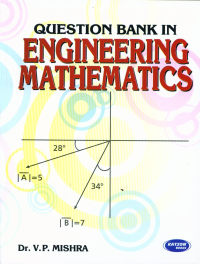•## Question Bank in Engineering Mathematics

In Stock
ISBN 978-93-5014-162-5 Dr. V.P. Mishra S.K. Kataria & Sons 2nd 2011 2009 875 Print In Stock
Rs 365.00Rs 329.00

#### CONTENTS

• Complex Numbers: de Moivre’s Theorem • Infinite Series: Convergence and Divergence • Successive Differentiation, Expansion of Functions and Approximate Calculations • Asymptotes and Curve Tracing • Mean Value Theorems and Curvature • Definite Integrals and Reduction Formulae • Applications of Integration • Matrices and their Applications • First Order Differential Equations and their Applications • Linear Differential Equations of Higher Order and Applications • Partial Differentiation • Applications of Partial Differentiation • Multiple Integrals • Vector Differentiation • Vector Integration • Functions of a Complex Variable–Analyticity and Transformation • Complex Integration • Laplace Transformation and its Applications • Fourier Series and Harmonic Analysis • BETA, GAMMA Functions and Series Solution of Differential Equations • Bessel’s Equation and Bessel Functions • Legendre’s Equation, Legendre Polynomials • First Order Partial Differential Equations • Partial Differential Equations of Higher Order and Applications • Fourier Transforms and Applications • Error Analysis and Numerical Solution of Equations • Finite Differences and Interpolation • Numerical Differentiation and Integration • Difference Equation and Numerical Solution of Ordinary Differential Equations.

• Author : Dr. V.P. Mishra
• Publisher : S.K. Kataria & Sons
• Edition : 2nd 2011
• Publishing Year : 2009
• Total Pages : 875